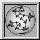Up: Geometry Forum Articles

# Hoops in Three-Space

Evelyn Sander
geometry.college, June 9, 1993.

A hoop is a geometric unit circle in three-space, or in other words, the result of applying a three dimensional rigid motion to the unit circle in the plane. Notice that a hoop is a circular curve and not a disk. Asimov considers what kind of three-dimensional regions one can fill with non-intersecting (but possibly interlocking) hoops. He is interested in the specific method of filling volumes with hoops, requiring that the hoops be placed in a continuous manner.

Here is a precise definition of continuity of hoops in a region of three space: One can specify a hoop using a center point and a normal direction. If hoops fill a region, then there is a map which assigns to each point in the region the hoop passing through that point. A continuous placement of hoops is one in which this map is continuous. (Technical note: The center of a hoop is a point in three space, R^3. The normal is a point on the unit sphere, S^2 or on the projective plane, P^2, depending on whether hoops are oriented or unoriented. Thus the map goes from R^3 to either R^3 x S^2 or R^3 x P^2.)

It is in fact possible to fill all of three-space with disjoint hoops. In 1964 J. Conway and H.T. Croft proved this using an Axiom of Choice argument. However, the placement of the hoops is not continuous or constructive, as is generally the case with the Axiom of Choice.

One can use a continuous placement of hoops to cover a "unit torus," meaning a torus of revolution whose core circle has radius one. (An example of this is the result of revolving the circle (x-1)^2 + y^2 = r^2 in R^2 about the z-axis of R^3. The shape of a unit torus is determined by the radius r < 1 of the circle being revolved.)

Notice the relationship between a unit torus and hoops; a unit torus is a surface of revolution around a hoop. In order to continuously fill a unit torus with disjoint hoops, each hoop must tilt so that it goes around the non unit circle of the torus exactly once as it goes around the unit circle of the torus.

Using this covering method for unit tori with (non unit) circles of all radii between zero and r, it is possible to continuously fill a solid radius r unit torus with disjoint hoops. Fattening the torus as much as possible without having the hoops intersect, one obtains a solid unit torus of radius one (by slight abuse of terminology). This means the torus has no hole in the center. Asimov terms this shape a "bialy," after a kind of bread similar to a bagel.

The bialy at least gives a lower bound of 2*(pi^2) for the possible connected volumes one can continuously fill with disjoint hoops. What about an upper bound? Asimov proved that for a set of hoops which fill a connected volume, any two hoops must link. Thus any volume continuously filled with hoops must fit inside a ball of radius three (whose volume is 36*pi). Although he does not yet have a least upper bound, it is clear that the restriction to continuous placement of hoops is indeed a severe restriction on the possible filled volume; it changes the possible region from all of three space to a region contained in a ball radius three!

Asimov is currently trying to extend his results on hoops. He is considering a variety of dimensions of geometric unit spheres in higher dimensional spaces.

Up: Geometry Forum ArticlesThe Geometry Center Home Page